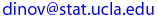Ivo Dinov
UCLA Statistics, Neurology, LONI
, Math/PIC
 Courses SOCR Ivo Dinov's Home SiteMap Software Contact

STAT 35
Winter  2005

Interactive and Computational Probability

Department of Statistics

## Instructor: Ivo Dinov

Homework 4
Due Date: Monday, Feb. 28, 2005

Please, submit your homework right after lecture on the due date. See the HW submission rules. On the front page include the following header.

• (HW_4_1) The pmf for X = the number of major defects on a randomly selected applicance of a certain type is given in the table below. Compute the following:
 x 0 1 2 3 4 p(x) 0.08 0.15 0.45 0.27 0.05
(a) E(X), the expected value of the R.V. X.
(b) V(X), the variance of X.
(c) SD(X), the standard deviation of X.
(d) V(X), using the shortcut formula, V(X)  =  E(X2 ) − µ2. Should equal the answer in part (b).

• (HW_4_2) An appliance dealer sells three different models of upright freezers having 13.5 ft3, 15.9 ft3, and 19.1 ft3 (cubic feet) of storage space, respectively. Let X = the amount of storage space purchased by the next customer to buy a freezer. Suppose that X has pmf.
 x 13.5 15.9 19.1 p(x) 0.2 0.5 0.3

(a) Compute the first three moments of the random varible X, E(X), E(X2), E(X3), and V(X).
(b) If the price of a freezer having capacity X ft3 is 25X − 8.5, what is the expected price paid by the next customer to buy a freezer?
(c) What is the variance of the price 25X − 8.5 paid by the next customer? Interpret this value!
(d) Suppose that although the rated capacity of a freezer is X, the actual capacity is h(X) = X − 0.01X2, slightly under the advertised space. What is the expected actual capacity of the freezer purchased by the next customer?

• (HW_4_3)  Use the SOCR Binomial Coin Experiment to compare the theoretical and empirically observed mean and standard deviation of this experiment. Run 10 times the experiment with number-of-coins n=14 and P(Head)=p=0.3. How different are the population (theoretical) and the sample (observed) mean and standard deviation? How many runs of the 14-coin-toss experiment you need to do to get both of these differences within 10-2? If you do another 10 runs are these differences guaranteed to stay within this limit (do not refresh the experiment, do an extra 10 runs)? Explain in your own words!

• (HW_4_4) Suppose you are randomly clipping coupons out of published materials. Each week before you go to the market you keep clipping coupons until you have 20 distinct coupons (some of the ones already clipped may be duplicates of others). Suppose there are 50 distinct vendors that advertise and print coupons in your  local media. Using the SOCR Coupon Collector Experiment do the following:
• Sellect the proper values for the parameters m (population-size) and k (numer of distinct objects desired). Look at the corresponding distribution for the random valiable W, which represents the total number of coupons clipped by shopping time.Without running any experiments, just look at the blue model distribution graph and the discrete model distribution table for W below, to estimate the odds that you will need to clip at least 35 coupons to get 20 distinct ones, as desired.
• Now, run 1,000 experiments in auto-mode. In what proportion of these you had to clip at least 35 coupons to get 20 distinct ones, as desired?
• What is the discrepancy between the theoretical probability and the emperically observed odds of having to clip at most 24 coupons, in the above 1,000 experiments?

 Ivo Dinov's Home http://www.stat.ucla.edu/~dinov Visitor number, since Jan. 01, 2002 © I.D.Dinov 1997-2005
Last modified on by